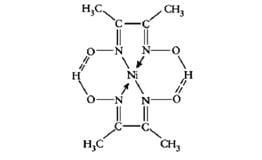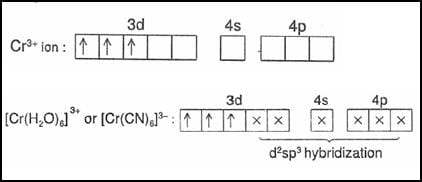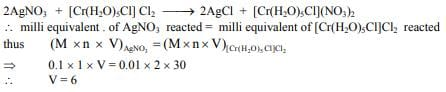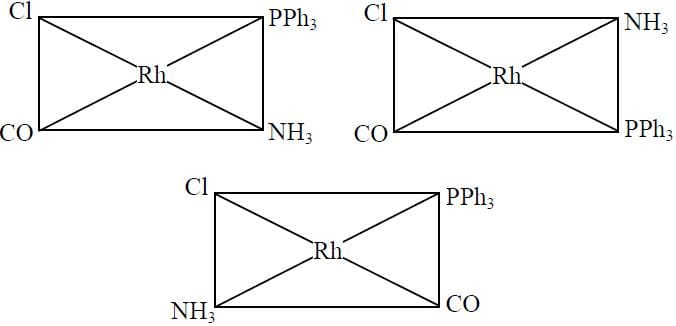Test: Coordination Chemistry- 1

# Test: Coordination Chemistry- 1

Test Description

## 20 Questions MCQ Test Inorganic Chemistry | Test: Coordination Chemistry- 1

Test: Coordination Chemistry- 1 for Chemistry 2023 is part of Inorganic Chemistry preparation. The Test: Coordination Chemistry- 1 questions and answers have been prepared according to the Chemistry exam syllabus.The Test: Coordination Chemistry- 1 MCQs are made for Chemistry 2023 Exam. Find important definitions, questions, notes, meanings, examples, exercises, MCQs and online tests for Test: Coordination Chemistry- 1 below.
Solutions of Test: Coordination Chemistry- 1 questions in English are available as part of our Inorganic Chemistry for Chemistry & Test: Coordination Chemistry- 1 solutions in Hindi for Inorganic Chemistry course. Download more important topics, notes, lectures and mock test series for Chemistry Exam by signing up for free. Attempt Test: Coordination Chemistry- 1 | 20 questions in 25 minutes | Mock test for Chemistry preparation | Free important questions MCQ to study Inorganic Chemistry for Chemistry Exam | Download free PDF with solutions
 1 Crore+ students have signed up on EduRev. Have you?
Test: Coordination Chemistry- 1 - Question 1

### Which of the following represents a chelating ligand

Detailed Solution for Test: Coordination Chemistry- 1 - Question 1

Because DMG is a polydentate ligand that binds the central atom nickel forming a ring-like structure.Test: Coordination Chemistry- 1 - Question 2

### The complex compounds which result from the coordination of carbon monoxide are known as

Detailed Solution for Test: Coordination Chemistry- 1 - Question 2

Metal carbonyls are coordination complexes of transition metals with carbon monoxide ligands. Metal carbonyls are useful in organic synthesis and as catalysts or catalyst precursors in homogeneous catalysis,

Fe(CO)5, Ni(CO)4 etc.

Test: Coordination Chemistry- 1 - Question 3

### Which of the following cannot act as a ligand?

Detailed Solution for Test: Coordination Chemistry- 1 - Question 3
• NHis a neutral ligand.
• NOis a cationic ligand.
• Cl– is an anionic ligand.
• However, BFis not a ligand as it does not have lone pairs to donate and can not share a pair of electrons.

Test: Coordination Chemistry- 1 - Question 4

As a ligand Cl is:

Detailed Solution for Test: Coordination Chemistry- 1 - Question 4

Only a σ–donor

Explanation: A ligand is a molecule or ion that binds to a central metal atom in a coordination complex. Cl- is a halide ion that can act as a ligand, and it predominantly forms σ-bonds due to the overlap of its filled p-orbitals with the empty orbitals on the metal atom. Cl- does not have any empty orbitals to accept electrons from the metal atom (σ-acceptor) nor does it have the ability to form π-bonds (π-donor). Therefore, as a ligand, Cl- is only a σ-donor.

Test: Coordination Chemistry- 1 - Question 5

In the complexes [Fe(CN)6]3– and [Co(en)3]3+, the coordination number of iron and cobalt are respectively (en = ethylenediamine):

Detailed Solution for Test: Coordination Chemistry- 1 - Question 5
• In 1st compound, CN is a unidentate ligand and hence it will surround the Fe atom from 6 different sides.
• On the other hand, the 2nd compound contains bidentate ligand so each (en) will have two different sites of donating electrons and hence it required 3 (en) atoms for surrounding Co atom from 6 different sides.
Hence A is correct.
Test: Coordination Chemistry- 1 - Question 6

The oxidation number, coordination number and magnetic moment in the following complex, [Cr(C2O4)2 (NH3)2]- is:

Detailed Solution for Test: Coordination Chemistry- 1 - Question 6
• Oxidation number:
x + (-2 x 2) + 0 x 2 = -1
x = +3
• The coordination number is 6 because C2O42-  is a bidentate ligand and NH3 is monodentate.
• Magnetic moment = √n(n+2)
where n is no. of unpaired electrons.
For Cr, n = 3 so the Magnetic moment will be √15 BM.
Test: Coordination Chemistry- 1 - Question 7

Following are the transition metal ions of 3d series: Ti4+,V2+,Mn3+,Cr3+
(Atomic numbers: Ti=22,V=23,Mn=25,Cr=24)
Which ion is most stable?

Detailed Solution for Test: Coordination Chemistry- 1 - Question 7

Ti4+ is most stable as it has completely filled 3p orbital.

Test: Coordination Chemistry- 1 - Question 8

Octahedral complex of Cr (III) will be:

Detailed Solution for Test: Coordination Chemistry- 1 - Question 8

The correct option is C

• Six empty orbitals (two d, one s and three p) are available, so whether the ligand is weak (H2O) or strong (CN)
• The six orbitals hybridize to give six equivalent d2sp3 hybrid orbitals.
• Hence, in both [Cr(H2O)6]3+
and [Cr(CN)6]3− ions chromium is in a state of d2sphybridization.Test: Coordination Chemistry- 1 - Question 9

Which of the following is a spin paired complex ion?

Detailed Solution for Test: Coordination Chemistry- 1 - Question 9
• In [Co(NH3)6]3+, the oxidation state of cobalt is +3. Ammonia is a strong field ligand so it pairs up 4 unpaired electrons and frees up 2,3−d orbitals.
• These 3−d orbitals are involved in hybridisation with one 4s and three 4p orbitals forming an inner orbital complex, so hybridisation of [Co(NH3)6]3+ is d2sp3.
Test: Coordination Chemistry- 1 - Question 10

Compound ‘X’ has the molecular formula CrCl2Br.6H2O. It can show which type of isomerism:
(I) Hydrate isomerism
(II) Ionization isomerism
(III) Geometrical isomerism
(IV) Optical isomerism

Detailed Solution for Test: Coordination Chemistry- 1 - Question 10

Correct option is A)

From the formula CrCl2​Br.6H2​O following complexes can be derived
- [Cr(H2​O)6​]BrCl2​[CrBr(H2​O)5​]Cl2​.H2​O
- [CrCl(H2​O)5​]BrCl.H2​O[CrCl2​(H2​O)4​]Br.2H2​O
- [CrBrCl(H2​O)4​]Cl.2H2​O.

Test: Coordination Chemistry- 1 - Question 11

What is the change in the oxidation state of cobalt in the following reaction?

[Co(NH3)4 Cl2]+ + H2O → [Co(NH3)4 (H2O)Cl]2+ + Cl

Detailed Solution for Test: Coordination Chemistry- 1 - Question 11

In [Co(NH3)4Cl2]

• (O.S. of Co + charge on ligands) = +1
• ∴ (O.S. of Co + 4 × charge of NH3 molecule + 2 × charge of Cl ion) = +1
• ∴ O.S. of Co + 4 × 0 + 2 × –1 = +1
• Oxidation state of Co = +3

In [Co(NH3)4 (H2O)Cl]2+

• (O.S. of Co + charge on ligands) = +2
• (O.S. of Co + 4 × charge of NH3 molecule + charge of H2O + charge of Cl ion) = +2
• ∴ O.S. of Co + 4 × 0 + 0 –1 = +2
• Oxidation state of Co = +3

Hence, Oxidation State of Cobalt does not change.

Test: Coordination Chemistry- 1 - Question 12

The first-row transition metal complexes having tetrahedral geometry are high–spin due to:

Detailed Solution for Test: Coordination Chemistry- 1 - Question 12

The correct option is B. Δt<P

• Tetrahedral complexes always show high spin complexes i.e. no pairing of electrons, because in tetrahedral complexes the coordination number of metal is four only, thus crystal field splitting is less in tetrahedral complexes.
• Hence, pairing energy is more compared to the crystal field splitting energy.
Test: Coordination Chemistry- 1 - Question 13

The zero magnetic moment of octahedral K2NiF6 is due to:

Detailed Solution for Test: Coordination Chemistry- 1 - Question 13

Oxidation state of Ni in [NiF6]2- :
x − 6 = −2
x = + 4
In +4 oxidation state, fluoride also behaves as a strong field ligand.
⇒ low spin d6 Ni(IV) complex.
⇒ unpaired electrons = 0.
⇒ zero magnetic moment.

Test: Coordination Chemistry- 1 - Question 14

What is the spin only magnetic moment value in (Bohr Magneton units) of Cr(CO)6?

Detailed Solution for Test: Coordination Chemistry- 1 - Question 14
• The electron configuration is [Ar]3d54s1.
• We have to accommodate the 6 Ligands and the fact that CO is a strong ligand, this results in d2sp3 hybridization.
• Therefore, there are no unpaired electrons in Cr(CO)6. So n = 0. Thus, the spin only magnetic moment is also 0.
Test: Coordination Chemistry- 1 - Question 15

The volume (in mL) of 0.1 M AgNO3 required for complex precipitation of chloride ions present in 30 mL of 0.01 M solution of [Cr(H2O)5Cl]Cl2, as silver chloride is close to:

Detailed Solution for Test: Coordination Chemistry- 1 - Question 15Test: Coordination Chemistry- 1 - Question 16

Which of the following elements do not form a complex with EDTA?

Detailed Solution for Test: Coordination Chemistry- 1 - Question 16

Be is the only group 2 element that does not form a stable complex with [EDTA]4−⋅Mg2+ and Ca2+ have the greatest tendency to form complexes with [EDTA]4−.

Test: Coordination Chemistry- 1 - Question 17

When EDTA solution is added to Mg2+ ion solution, then which of the following statements is not true?

Detailed Solution for Test: Coordination Chemistry- 1 - Question 17
• When EDTA solution is added to the Mg2+ ion solution, all six-coordinate sites of Mg2+ are occupied, and a colourless [Mg−EDTA]2− chelate is formed. This reaction also results in the lowering of the pH of the solution.
• So, the correct answer is 'Four coordinates sites of Mg2+ are occupied by EDTA and the remaining two sites are occupied by water molecules.'
Test: Coordination Chemistry- 1 - Question 18

The total number of geometrical isomers for the complex [RhCl(CO)(PPh3)(NH3)] is:

Detailed Solution for Test: Coordination Chemistry- 1 - Question 18

The total number of geometrical isomers for the complex [RhCl(CO)(PPh3​)(NH3)] is since it is a square planar.Test: Coordination Chemistry- 1 - Question 19

Which one the following octahedral complexes will be distorted:

Detailed Solution for Test: Coordination Chemistry- 1 - Question 19
• [Cr(H2O)6]2+ is distorted because of more number of electrons i.e. 4 electrons (electron-electron repulsion).
• But in the case of Mn & Fe both of them has 3d5 configuration which is more stable & decreases the chances of distortion.
• In the case of Cr3+ less number of electrons are present, so, less electron-electron repulsion hence lesser will be the distortion.
• When there is unsymmetrical filling of electron in Eg orbital occur, the shape will distorted e.g. in the case of d4. This is known as Jahn-Teller distortion.
Hence A is correct.
Test: Coordination Chemistry- 1 - Question 20

The compound which exhibits Jahn-Teller distortion is:

Detailed Solution for Test: Coordination Chemistry- 1 - Question 20

The correct option is B

• The complexes in which the metal ion have a unsymmetrical eg or t2​g orbital shows Jahn-taller distortions.
• In the complex [Cu(H2​O)6​]2+ the configuration is  t2​g6eg3 i.e eg orbital is unsymmetrical and hence will show Jahn-Taller distortions.

## Inorganic Chemistry

43 videos|89 docs|37 tests
Information about Test: Coordination Chemistry- 1 Page
In this test you can find the Exam questions for Test: Coordination Chemistry- 1 solved & explained in the simplest way possible. Besides giving Questions and answers for Test: Coordination Chemistry- 1, EduRev gives you an ample number of Online tests for practice

## Inorganic Chemistry

43 videos|89 docs|37 tests(Scan QR code)